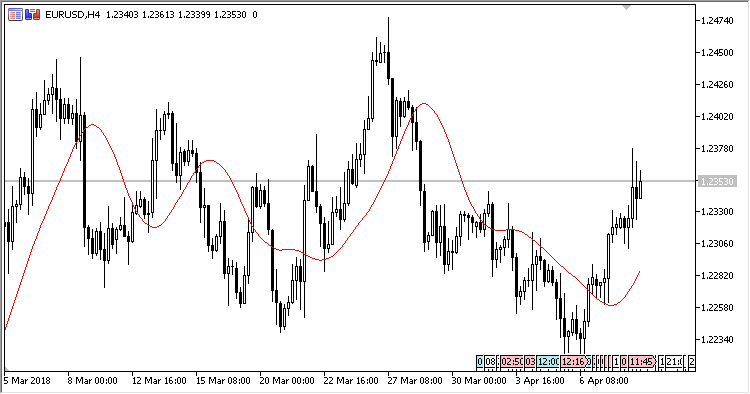Interesting script?
So post a link to it -
let others appraise it

You liked the script? Try it in the MetaTrader 5 terminal# TriMA - indicator for MetaTrader 5

Views:
1607
Rating:
Published:
2018.06.06 14:02

Triangular Moving Average.

Calculation:

TriMA[i] = (MVA(i,N) + MVA(i-1,N) + … + MVA(i-N+1,N))/N

where

```MVA(i,N) – a simple moving average (SMA),
N=(Period+1)/2
```

The indicator has two input parameters:

• Period - period;
• Applied price - price used for calculations.Translated from Russian by MetaQuotes Software Corp.
Original code: https://www.mql5.com/ru/code/20437Trailing_Stop_Level

An indicator of StopLoss levels.Simple_Support_Resistance

An indicator of current support/resistance levels.Dominant_Color

An indicator of the dominant direction of closing of bars.ILRS_MA

Integral of Linear Regression Slope (ILRS MA).